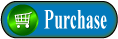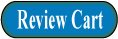`American Press `
`Academic, Professional, Technical, Trade, Research Books & Journals... `

americanpress@flash.net        617- 247- 0022        75 State Street #100        Boston, MA 02109

### Home  |   Subjects  |   Authors   |   Titles  |  ISBN's   |   Journals   |   Ordering   |   Writing  |  Contact

 INTERMEDIATE ALGEBRAby JOHN SCOTT 5th edition, 304 pages, \$26.95 ISBN 9780-89641-184-5  Designed for use at the college level, This text stresses the classical versus the modern math approach to algebra. The emphasis throughout the text is on learning the "how" of algebraic procedures rather than the "why." A large number of detailed examples appear in each chapter to aid in the reviewing process. One or more sets of problems are included in each chapter. This edition includes an Appendix on Rectangular Coordinates. SOLUTIONS MANUAL TO SCOTT'S INTERMEDIATE ALGEBRAby WILLIAM ECHOLS and ERNEST LOWERY 5th edition, 248 pages, \$19.95 ISBN 978-0-89641-195-1This manual contains detailed solutions to the odd-numbered exercises listed in Intermediate Algebra, Fifth Edition. Although only one solution is shown for each problem, a variety of methods may be used as many exercises can be worked in more than one way. Emphasis is placed on solutions rather than answers. The answers to all the odd-numbered problems are in the answer section of John B. Scott's Intermediate Algebra. Contents Chapter 1: FIRST CONCEPTS Introduction Signed Numbers Signed Number Arithmetic Problems Exponents Exponential Laws Scientific Notation Problems Chapter 2: BASIC ALGEBRAIC CONSIDERATIONS Introduction The Vocabulary of Algebra Numerical Substitutions Algebraic Addition and Subtraction Algebraic Products Algebraic Quotients Symbols of Grouping Problems Chapter 3: FACTORING ALGEBRAIC EXPRESSIONS Introduction Common Monomial Factoring The Difference of Two Squares Sum and Difference of Two Cubes Perfect Square Trinomials General Trinomial Factoring Factoring by Grouping. Problems Chapter 4: ALGEBRAIC FRACTIONS Introduction Equivalent Fractions Sign Considerations Multiplication and Division of Fractions Least Common Multiple Addition and Subtraction of Fractions Complex Fractions Problems Chapter 5: RADICAL AND EXPONENTS Introduction Rational and Irrational Numbers Roots Fractional Exponents Radical Simplification Arithmetic of Radicals Radicals of Differing Indices Problems Chapter 6: EQUATIONS OF FIRST DEGREE Introduction Postulates for Solving Equations The Linear Equation Extraneous Solutions Equations Involving Radicals Literal Equations Problems Chapter 7: STATED PROBLEMS Introduction General Suggestions Integer Problems Age Problems Uniform Motion Problems Mixture Problems Miscellaneous Problems Problems Chapter 8: THE COMPLEX NUMBER SYSTEM Introduction Powers of i Complex Numbers Complex Number Operations Problems Chapter 9: EQUATIONS OF SECOND DEGREE Introduction Solving the Incomplete Quadratic Equation Factoring the Complete Quadratic Equation Completing the Square The Quadratic Formula Equations Reducible to Quadratics Applied Problems Problems APPENDIX: RECTANGULAR COORDINATES ANSWERS TO SELECTED EXERCISES AND PROBLEMS About the Authors Dr. John B. Scott teaches mathematics for the Houston Community College System. He received his B.A. in Math from Hiram College, Hiram, Ohio, his M.A. in Math from Case Western Reserve University in Cleveland, and holds a Ph.D. in Math from the University of Houston. John spent four years with Texaco Research Laboratories as a Senior Mathematician, and has also co-authored Mathematics for Health Sciences. He is a member of the American Mathematical Society and the Mathematical Association of America. William A. Echols and Ernest Lowrey are both instructors of mathematics for the Houston Community College System in Houston, Texas.

 ORDER DIRECT AND SAVE! This book will be a great reference to your professional library! Ask your libraries to order books of interest. Students, professionals, teachers and libraries receive our best discount.

Home | Subjects | Authors | Titles | ISBN's | Journals | Ordering | Writing | Contact